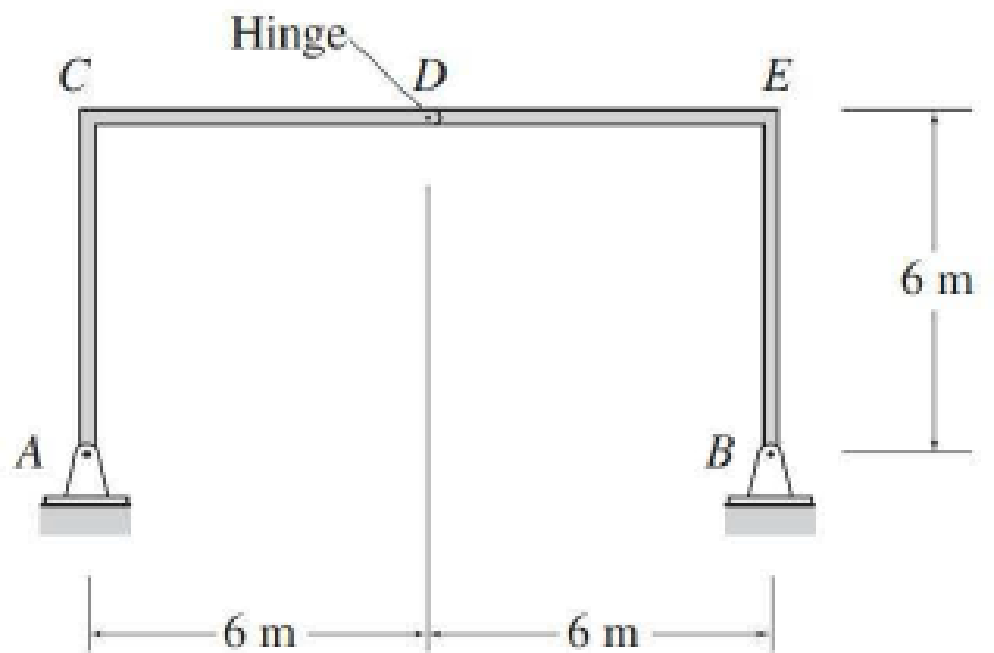# 8.35 and 8.36 Draw the influence lines for the horizontal and vertical reactions at supports A and B of the frames shown in Figs. P8.35 and P8.36. FIG. P8.35

#### Solutions

Chapter
Section
Chapter 8, Problem 35P
Textbook Problem
1 views

## 8.35 and 8.36 Draw the influence lines for the horizontal and vertical reactions at supports A and B of the frames shown in Figs. P8.35 and P8.36.FIG. P8.35To determine

Draw the influence lines for the vertical reactions at supports A and B.

### Explanation of Solution

Calculation:

Apply a 1 kN unit moving load at a distance of x from left end C.

Sketch the free body diagram of frame as shown in Figure 1.

Refer Figure 1.

Find the vertical equation of support reaction (Ay) at A using equilibrium equation:

Consider moment equilibrium at point B.

Consider clockwise moment as positive and anticlockwise moment as negative

Sum of moment at point B is zero.

ΣMB=0Ay(12)1(12x)=012Ay=12xAy=1x12 (1)

Find the influence line ordinate of Ay at C using Equation (1).

Substitute 0 for x in Equation (1).

Ay=1012=1kN/kN

Similarly calculate the influence line ordinate of Ay at various points on the beam and summarize the values in Table 1.

 x (m) Points Influence line ordinate of Ay(kN/kN) 0 C 1 6 D 12 12 E 0

Sketch the influence line diagram for the vertical reaction at support A using Table 1 as shown in Figure 2.

Refer Figure 1.

Find the equation of support reaction (By) at B using force equilibrium equation:

Consider force equilibrium along vertical.

Consider the upward force as positive (+) and downward force as negative ().

Ay+By=1

Substitute 1x12 for Ay.

1x12+By=1By=11+x12By=x12 (2)

Find the influence line ordinate of By at C using Equation (1).

Substitute 0 for x in Equation (2).

By=012=0

Similarly calculate the influence line ordinate of By at various points on the beam and summarize the values in Table 2.

 x (m) Points Influence line ordinate of By(kN/kN) 0 C 0 6 D 12 12 E 1

Sketch the influence line diagram for the vertical reaction at support E using Table 2 as shown in Figure 3.

Refer Figure 1.

Apply 1 kN load at just left of D. (0x6m)

Consider the section DE.

Find the horizontal support reaction (Bx) at B using moment equilibrium equation:

Apply moment equilibrium at D from B.

Consider the clockwise moment as negative and counter clockwise moment as positive.

ΣMD=0Bx(6)By(6)=0Bx=By

Substitute x12 for By.

Bx=x12(+)

Apply 1 kN load at just right of D. (6mx12m)

Consider the section DE.

Find the horizontal support reaction (Bx) at B using moment equilibrium equation:

Apply moment equilibrium at D from B

### Still sussing out bartleby?

Check out a sample textbook solution.

See a sample solution

#### The Solution to Your Study Problems

Bartleby provides explanations to thousands of textbook problems written by our experts, many with advanced degrees!

Get Started

Find more solutions based on key concepts
For the ideal gas law given in Problem 6.31, if the units of P, V, m, and T are expressed in lbf/ft2, ft3, lbm,...

Engineering Fundamentals: An Introduction to Engineering (MindTap Course List)

Enhanced Discovering Computers 2017 (Shelly Cashman Series) (MindTap Course List)

How is above base speed obtained?

Electric Motor Control

What is the smallest graduation on a metric micrometer with a vernier scale?

Precision Machining Technology (MindTap Course List)

If your motherboard supports ECC DDR3 memory, can you substitute non-ECC DDR3 memory?

A+ Guide to Hardware (Standalone Book) (MindTap Course List)